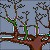## Numerical Example of Linear Discriminant Analysis (LDA)

Here is an example of LDA. We are going to solve linear discriminant using MS excel. You can download the worksheet companion of this numerical example here.

Factory "ABC" produces very expensive and high quality chip rings that their qualities are measured in term of curvature and diameter. Result of quality control by experts is given in the table below.

 Curvature Diameter Quality Control Result 2.95 6.63 Passed 2.53 7.79 Passed 3.57 5.65 Passed 3.16 5.47 Passed 2.58 4.46 Not Passed 2.16 6.22 Not Passed 3.27 3.52 Not Passed

As a consultant to the factory, you get a task to set up the criteria for automatic quality control. Then, the manager of the factory also wants to test your criteria upon new type of chip rings that even the human experts are argued to each other. The new chip rings have curvature 2.81 and diameter 5.46.

Can you solve this problem by employing Discriminant Analysis?

Solutions

When we plot the features, we can see that the data is linearly separable. We can draw a line to separate the two groups. The problem is to find the line and to rotate the features in such a way to maximize the distance between groups and to minimize distance within group.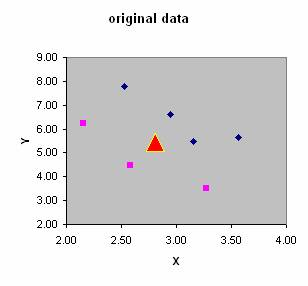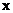= features (or independent variables) of all data. Each row (denoted by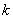) represents one object; each column stands for one feature.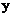= group of the object (or dependent variable) of all data. Each row represents one object and it has only one column.

In our example,and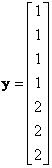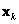= data of row. For example,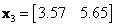,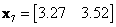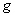= number of groups in. In our example,= 2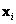= features data for group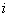. Each row represents one object; each column stands for one feature. We separateinto several groups based on the number of category in.,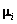= mean of features in group, which is average of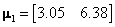,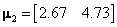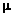= global mean vector, that is mean of the whole data set.

In this example,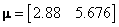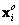= mean corrected data, that is the features data for group,, minus the global mean vector,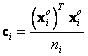= covariance matrix of group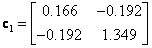,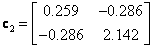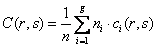= pooled within group covariance matrix. It is calculated for each entry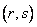in the matrix. In our example,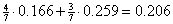,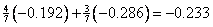and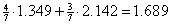, therefore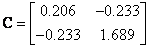The inverse of the pooled covariance matrix is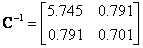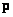= prior probability vector (each row represent prior probability of group). If we do not know the prior probability, we just assume it is equal to total sample of each group divided by the total samples, that is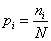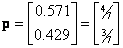Discriminant function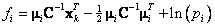We should assign objectto groupthat has maximum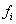The results of our computation are given in MS Excel as shown in the figure below.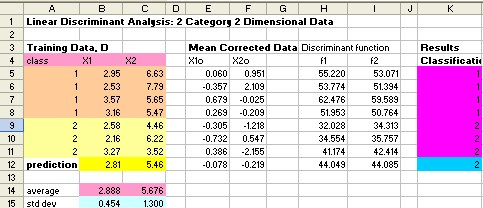The discriminant function is our classification rules to assign the object into separate group. If we input the new chip rings that have curvature 2.81 and diameter 5.46, reveal that it does not pass the quality control.

Transforming all data into discriminant function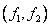we can draw the training data and the prediction data into new coordinate. The discriminant line is all data of discriminant function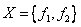and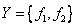. In MS Excel, you can hold CTRL key wile dragging the second region to select both regions.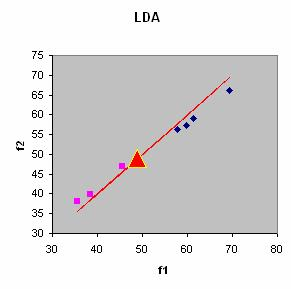Preferable reference for this tutorial is

Teknomo, Kardi (2015) Discriminant Analysis Tutorial. http://people.revoledu.com/kardi/ tutorial/LDA/